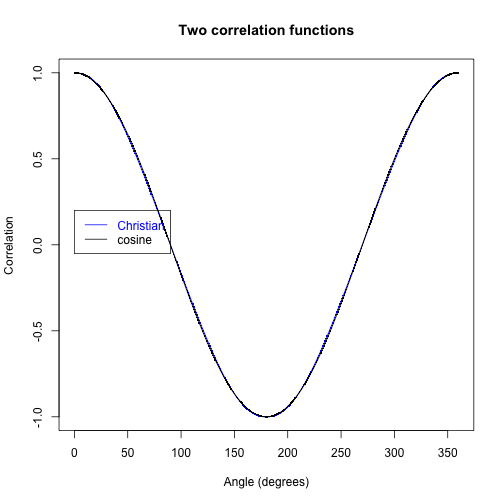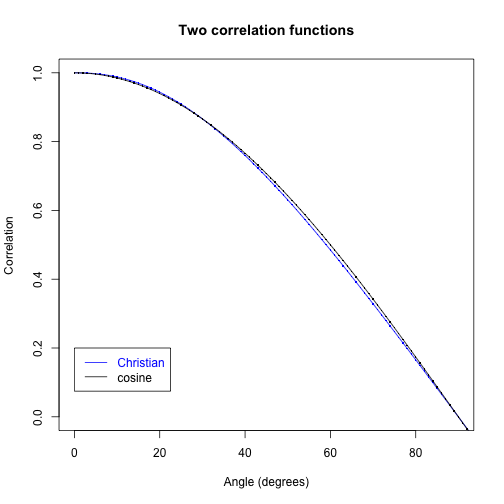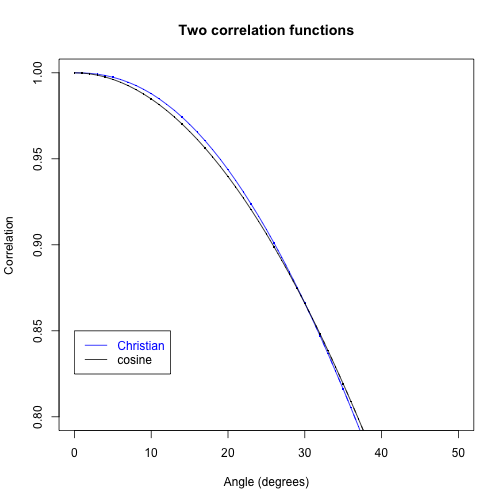### zen.R

richard — Feb 28, 2014, 7:41 PM

``````## A simulation of Joy Christian's 3-sphere model for the EPR-Bohm

## S^2 version (aka 3-D: think of S^2 as subset of R^3)

## This version has been adapted from Richard Gill's optimized version of
## Michel Fodje's original simulation, http://rpubs.com/gill1109/EPRB3opt.
## All these simulations are inspired by the simulation of Joy Christian's
## 3-sphere model by Chantal Roth, https://github.com/chenopodium/JCS2.

## The present S^2 version is similar to Michel's, but with uniform random
## points on S^2 (subset R^3) instead of uniform random points on S^1.

set.seed(9875)

## For reproducibility. Replace integer seed by your own, or delete this line
## and let your computer dream up one for you (system time + process ID).

## Uniform random points on sphere generated using 'trig method' (method 3)
## of Dave Seaman: http://www.math.niu.edu/~rusin/known-math/96/sph.rand
## http://mathforum.org/kb/message.jspa?messageID=393612 See
## http://rpubs.com/gill1109/13340 for an R illustration

## (a) Choose z uniformly distributed in [-1,1].  (b) Choose t uniformly
## distributed on [0, 2*pi).  (c) Let r = sqrt(1-z^2).  (d) Let x = r *
## cos(t).  (e) Let y = r * sin(t).

## Since the measurement directions are all in the equatorial plane, only z
## and x have been generated and treated as x and y

## Measurement angles for setting 'a': directions in the equatorial plane

angles <- seq(from = 0, to = 360, by = 1) * 2 * pi/360

K <- length(angles)
corrs <- numeric(K)  ## Container for correlations
Ns <- numeric(K)  ## Container for number of states

beta <- 0 * 2 * pi/360  ## Measurement direction 'b' fixed, in equatorial plane

M <- 10^7  ## Sample size. Next, try 10^7 or even 10^8 ...

## Follow Gill using the same, single sample of 'M' realizations of hidden
## states for all measurement directions. This saves a lot of time, and
## reduces variance when we look at *differences*.

z <- runif(M, -1, 1)
t <- runif(M, 0, 2 * pi)
r <- sqrt(1 - z^2)
x <- r * cos(t)

e <- rbind(z, x)  ## 2 x M matrix
## The M columns of e represent the x and y coordinates of M uniform random
## points on the sphere S^2

s <- rbeta(M, 1.29, 4.56) ## Zen's improved solution

b <- c(cos(beta), sin(beta))  ## Measurement vector 'b'

## Loop through measurement vectors 'a' (except last = 360 degrees = first)

for (i in 1:(K - 1)) {
alpha <- angles[i]
a <- c(cos(alpha), sin(alpha))  ## Measurement vector 'a'
ca <- colSums(e * a)  ## Inner products of cols of 'e' with 'a'
cb <- colSums(e * b)  ## Inner products of cols of 'e' with 'b'
good <- abs(ca) > s & abs(cb) > s  ## Select the 'states'
N <- sum(good)
corrs[i] <- sum(sign(ca[good]) * sign(cb[good]))/N
Ns[i] <- N
}

corrs[K] <- corrs
Ns[K] <- Ns

plot(angles * 180/pi, corrs, type = "l", col = "blue", main = "Two correlation functions",
xlab = "Angle (degrees)", ylab = "Correlation")
points(angles * 180/pi, corrs, col = "blue", pch = ".", cex = 2)
lines(angles * 180/pi, cos(angles), col = "black")
points(angles * 180/pi, cos(angles), col = "black", pch = ".", cex = 2)

legend(x = 0, y = 0.2, legend = c("Christian", "cosine"), text.col = c("blue",
"black"), lty = 1, col = c("blue", "black"))
````````````

plot(angles * 180/pi, corrs, type = "l", col = "blue", xlim = c(0, 90), ylim = c(0,
1), main = "Two correlation functions", xlab = "Angle (degrees)", ylab = "Correlation")
points(angles * 180/pi, corrs, col = "blue", pch = ".", cex = 2)
lines(angles * 180/pi, cos(angles), col = "black")
points(angles * 180/pi, cos(angles), col = "black", pch = ".", cex = 2)

legend(x = 0, y = 0.2, legend = c("Christian", "cosine"), text.col = c("blue",
"black"), lty = 1, col = c("blue", "black"))
````````````

plot(angles * 180/pi, corrs, type = "l", col = "blue", xlim = c(0, 50), ylim = c(0.8,
1), main = "Two correlation functions", xlab = "Angle (degrees)", ylab = "Correlation")
points(angles * 180/pi, corrs, type = "b", col = "blue", pch = ".", cex = 2)
lines(angles * 180/pi, cos(angles), col = "black")
points(angles * 180/pi, cos(angles), type = "b", col = "black", pch = ".", cex = 2)
legend(x = 0, y = 0.85, legend = c("Christian", "cosine"), text.col = c("blue",
"black"), lty = 1, col = c("blue", "black"))
````````````

plot(angles * 180/pi, corrs - cos(angles), type = "l")
````````````
max(abs(corrs - cos(angles)))
``````
`````` 0.01505
``````